x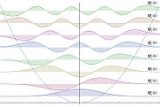Quantum harmonic oscillatorOverview

The quantum harmonic oscillator is the quantum-mechanical
Quantum mechanics
Quantum mechanics, also known as quantum physics or quantum theory, is a branch of physics providing a mathematical description of much of the dual particle-like and wave-like behavior and interactions of energy and matter. It departs from classical mechanics primarily at the atomic and subatomic...

analog of the classical harmonic oscillator
Harmonic oscillator
In classical mechanics, a harmonic oscillator is a system that, when displaced from its equilibrium position, experiences a restoring force, F, proportional to the displacement, x: \vec F = -k \vec x \, where k is a positive constant....

. Because an arbitrary potential can be approximated as a harmonic potential at the vicinity of a stable equilibrium point, it is one of the most important model systems in quantum mechanics. Furthermore, it is one of the few quantum-mechanical systems for which an exact, analytical solution is known.

The Hamiltonian
Hamiltonian (quantum mechanics)
In quantum mechanics, the Hamiltonian H, also Ȟ or Ĥ, is the operator corresponding to the total energy of the system. Its spectrum is the set of possible outcomes when one measures the total energy of a system...

of the particle is: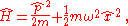where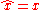is the position operator
Position operator
In quantum mechanics, the position operator is the operator that corresponds to the position observable of a particle. Consider, for example, the case of a spinless particle moving on a line. The state space for such a particle is L2, the Hilbert space of complex-valued and square-integrable ...

, and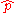is the momentum operator
Momentum operator
In quantum mechanics, momentum is defined as an operator on the wave function. The Heisenberg uncertainty principle defines limits on how accurately the momentum and position of a single observable system can be known at once...

, given byThe first term in the Hamiltonian represents the kinetic energy of the particle, and the second term represents the potential energy in which it resides.Applications of the harmonic oscillatorin modern physics only example>> thanke you very muchEncyclopedia
The quantum harmonic oscillator is the quantum-mechanical
Quantum mechanics
Quantum mechanics, also known as quantum physics or quantum theory, is a branch of physics providing a mathematical description of much of the dual particle-like and wave-like behavior and interactions of energy and matter. It departs from classical mechanics primarily at the atomic and subatomic...

analog of the classical harmonic oscillator
Harmonic oscillator
In classical mechanics, a harmonic oscillator is a system that, when displaced from its equilibrium position, experiences a restoring force, F, proportional to the displacement, x: \vec F = -k \vec x \, where k is a positive constant....

. Because an arbitrary potential can be approximated as a harmonic potential at the vicinity of a stable equilibrium point, it is one of the most important model systems in quantum mechanics. Furthermore, it is one of the few quantum-mechanical systems for which an exact, analytical solution is known.

### Hamiltonian and energy eigenstates

The Hamiltonian
Hamiltonian (quantum mechanics)
In quantum mechanics, the Hamiltonian H, also Ȟ or Ĥ, is the operator corresponding to the total energy of the system. Its spectrum is the set of possible outcomes when one measures the total energy of a system...

of the particle is:whereis the position operator
Position operator
In quantum mechanics, the position operator is the operator that corresponds to the position observable of a particle. Consider, for example, the case of a spinless particle moving on a line. The state space for such a particle is L2, the Hilbert space of complex-valued and square-integrable ...

, andis the momentum operator
Momentum operator
In quantum mechanics, momentum is defined as an operator on the wave function. The Heisenberg uncertainty principle defines limits on how accurately the momentum and position of a single observable system can be known at once...

, given byThe first term in the Hamiltonian represents the kinetic energy of the particle, and the second term represents the potential energy in which it resides. In order to find the energy
Energy
In physics, energy is an indirectly observed quantity. It is often understood as the ability a physical system has to do work on other physical systems...

levels and the corresponding energy eigenstates, we must solve the time-independent Schrödinger equation
Schrödinger equation
The Schrödinger equation was formulated in 1926 by Austrian physicist Erwin Schrödinger. Used in physics , it is an equation that describes how the quantum state of a physical system changes in time....

,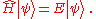We can solve the differential equation in the coordinate basis, using a spectral method
Spectral method
Spectral methods are a class of techniques used in applied mathematics and scientific computing to numerically solve certain Dynamical Systems, often involving the use of the Fast Fourier Transform. Where applicable, spectral methods have excellent error properties, with the so called "exponential...

. It turns out that there is a family of solutions. In the position basis they are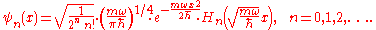The functions Hn are the Hermite polynomials
Hermite polynomials
In mathematics, the Hermite polynomials are a classical orthogonal polynomial sequence that arise in probability, such as the Edgeworth series; in combinatorics, as an example of an Appell sequence, obeying the umbral calculus; in numerical analysis as Gaussian quadrature; and in physics, where...

:The corresponding energy levels are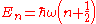.

This energy spectrum is noteworthy for three reasons. First, the energies are quantized, meaning that only discrete energy values (half-integer multiples of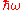) are possible; this is a general feature of quantum-mechanical systems when a particle is confined. Second, these discrete energy levels are equally spaced, unlike in the Bohr model
Bohr model
In atomic physics, the Bohr model, introduced by Niels Bohr in 1913, depicts the atom as a small, positively charged nucleus surrounded by electrons that travel in circular orbits around the nucleus—similar in structure to the solar system, but with electrostatic forces providing attraction,...

of the atom, or the particle in a box
Particle in a box
In quantum mechanics, the particle in a box model describes a particle free to move in a small space surrounded by impenetrable barriers. The model is mainly used as a hypothetical example to illustrate the differences between classical and quantum systems...

. Third, the lowest achievable energy (the energy of thestate, called the ground state
Ground state
The ground state of a quantum mechanical system is its lowest-energy state; the energy of the ground state is known as the zero-point energy of the system. An excited state is any state with energy greater than the ground state...

) is not equal to the minimum of the potential well, but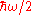above it; this is called zero-point energy
Zero-point energy
Zero-point energy is the lowest possible energy that a quantum mechanical physical system may have; it is the energy of its ground state. All quantum mechanical systems undergo fluctuations even in their ground state and have an associated zero-point energy, a consequence of their wave-like nature...

. Because of the zero-point energy, the position and momentum of the oscillator in the ground state are not fixed (as they would be in a classical oscillator), but have a small range of variance, in accordance with the Heisenberg uncertainty principle. The zero-point energy also has important implications in quantum field theory
Quantum field theory
Quantum field theory provides a theoretical framework for constructing quantum mechanical models of systems classically parametrized by an infinite number of dynamical degrees of freedom, that is, fields and many-body systems. It is the natural and quantitative language of particle physics and...

and quantum gravity
Quantum gravity
Quantum gravity is the field of theoretical physics which attempts to develop scientific models that unify quantum mechanics with general relativity...

.

Note that the ground state probability density is concentrated at the origin. This means the particle spends most of its time at the bottom of the potential well, as we would expect for a state with little energy. As the energy increases, the probability density becomes concentrated at the classical "turning points", where the state's energy coincides with the potential energy. This is consistent with the classical harmonic oscillator, in which the particle spends most of its time (and is therefore most likely to be found) at the turning points, where it is the slowest. The correspondence principle
Correspondence principle
In physics, the correspondence principle states that the behavior of systems described by the theory of quantum mechanics reproduces classical physics in the limit of large quantum numbers....

is thus satisfied.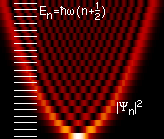The spectral method solution, though straightforward, is rather tedious. The "ladder operator
In linear algebra , a raising or lowering operator is an operator that increases or decreases the eigenvalue of another operator. In quantum mechanics, the raising operator is sometimes called the creation operator, and the lowering operator the annihilation operator...

" method, due to Paul Dirac
Paul Dirac
Paul Adrien Maurice Dirac, OM, FRS was an English theoretical physicist who made fundamental contributions to the early development of both quantum mechanics and quantum electrodynamics...

, allows us to extract the energy eigenvalues without directly solving the differential equation. Furthermore, it is readily generalizable to more complicated problems, notably in quantum field theory
Quantum field theory
Quantum field theory provides a theoretical framework for constructing quantum mechanical models of systems classically parametrized by an infinite number of dynamical degrees of freedom, that is, fields and many-body systems. It is the natural and quantitative language of particle physics and...

. Following this approach, we define the operators a and its adjoint
In mathematics, specifically in functional analysis, each linear operator on a Hilbert space has a corresponding adjoint operator.Adjoints of operators generalize conjugate transposes of square matrices to infinite-dimensional situations...

a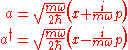The operator a is not Hermitian since it and its adjoint a are not equal.

We can also define a number operator N which has the following property: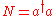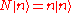The following commutator
Commutator
In mathematics, the commutator gives an indication of the extent to which a certain binary operation fails to be commutative. There are different definitions used in group theory and ring theory.-Group theory:...

s can be easily obtained by substituting the canonical commutation relation
Canonical commutation relation
In physics, the canonical commutation relation is the relation between canonical conjugate quantities , for example:[x,p_x] = i\hbar...

,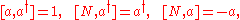And the Hamilton operator can be expressed as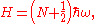so the eigenstate of N is also the eigenstate of energy.

The commutation properties yields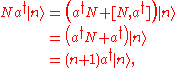and similarly,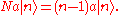This means that a acts on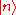to produce, up to a multiplicative constant,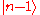, and a acts onto produce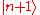. For this reason, a is called a "lowering operator", and a a "raising operator". The two operators together are called ladder operator
In linear algebra , a raising or lowering operator is an operator that increases or decreases the eigenvalue of another operator. In quantum mechanics, the raising operator is sometimes called the creation operator, and the lowering operator the annihilation operator...

s. In quantum field theory, a and a are alternatively called "annihilation" and "creation" operators because they destroy and create particles, which correspond to our quanta of energy.

Given any energy eigenstate, we can act on it with the lowering operator, a, to produce another eigenstate with-less energy. By repeated application of the lowering operator, it seems that we can produce energy eigenstates down to E = −∞. However, since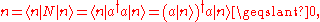the smallest eigen number is 0, and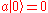.

In this case, subsequent applications of the lowering operator will just produce zero kets, instead of additional energy eigenstates. Furthermore, we have shown above that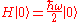Finally, by acting on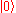with the raising operator and multiplying by suitable normalization factors, we can produce an infinite set of energy eigenstates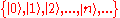, such that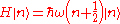which matches the energy spectrum which we gave in the preceding section.

Arbitrary eigenstate can be expressed in terms of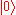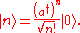Proof: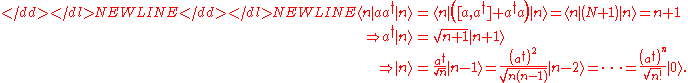### Natural length and energy scales

The quantum harmonic oscillator possesses natural scales for length and energy, which can be used to simplify the problem. These can be found by nondimensionalization. The result is that if we measure energy in units ofand distance in units of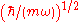, then the Schrödinger equation becomes: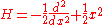,

and the energy eigenfunctions and eigenvalues become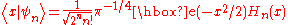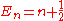where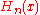are the Hermite polynomials
Hermite polynomials
In mathematics, the Hermite polynomials are a classical orthogonal polynomial sequence that arise in probability, such as the Edgeworth series; in combinatorics, as an example of an Appell sequence, obeying the umbral calculus; in numerical analysis as Gaussian quadrature; and in physics, where...

.

To avoid confusion, we will not adopt these natural units in this article. However, they frequently come in handy when performing calculations.

### Diatomic molecules

The vibrations of a diatomic molecule are an example of a two-body version of the quantum harmonic oscillator. In this case, the angular frequency is given by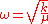where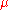is the reduced mass
Reduced mass
Reduced mass is the "effective" inertial mass appearing in the two-body problem of Newtonian mechanics. This is a quantity with the unit of mass, which allows the two-body problem to be solved as if it were a one-body problem. Note however that the mass determining the gravitational force is not...

and is determined by the mass of the two atoms.

## N-dimensional harmonic oscillator

The one-dimensional harmonic oscillator is readily generalizable to N dimensions, where N = 1, 2, 3, ... . In one dimension, the position of the particle was specified by a single coordinate
Coordinate system
In geometry, a coordinate system is a system which uses one or more numbers, or coordinates, to uniquely determine the position of a point or other geometric element. The order of the coordinates is significant and they are sometimes identified by their position in an ordered tuple and sometimes by...

, x. In N dimensions, this is replaced by N position coordinates, which we label x1, ..., xN. Corresponding to each position coordinate is a momentum; we label these p1, ..., pN. The canonical commutation relations between these operators are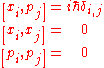.

The Hamiltonian for this system is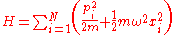.

As the form of this Hamiltonian makes clear, the N-dimensional harmonic oscillator is exactly analogous to N independent one-dimensional harmonic oscillators with the same mass and spring constant. In this case, the quantities x1, ..., xN would refer to the positions of each of the N particles. This is a convenient property of the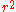potential, which allows the potential energy to be separated into terms depending on one coordinate each.

This observation makes the solution straightforward. For a particular set of quantum numbers {n} the energy eigenfunctions for the N-dimensional oscillator are expressed in terms of the 1-dimensional eigenfunctions as: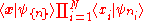In the ladder operator method, we define N sets of ladder operators,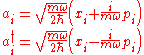.

By a procedure analogous to the one-dimensional case, we can then show that each of the ai and ai operators lower and raise the energy by ℏω respectively. The Hamiltonian is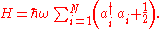This Hamiltonian is invariant under the dynamic symmetry group U(N) (the unitary group in N dimensions), defined by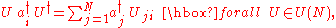where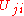is an element in the defining matrix representation of U(N).

The energy levels of the system are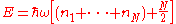.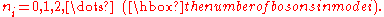As in the one-dimensional case, the energy is quantized. The ground state energy is N times the one-dimensional energy, as we would expect using the analogy to N independent one-dimensional oscillators. There is one further difference: in the one-dimensional case, each energy level corresponds to a unique quantum state. In N-dimensions, except for the ground state, the energy levels are degenerate, meaning there are several states with the same energy.

The degeneracy can be calculated relatively easily. As an example, consider the 3-dimensional case: Define n = n1 + n2 + n3. All states with the same n will have the same energy. For a given n, we choose a particular n1. Then n2 + n3 = n − n1. There are n − n1 + 1 possible groups {n2n3}. n2 can take on the values 0 to n − n1, and for each n2 the value of n3 is fixed. The degree of degeneracy therefore is: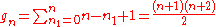Formula for general N and n [gn being the dimension of the symmetric irreducible nth power representation of the unitary group U(N)]: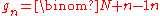The special case N = 3, given above, follows directly from this general equation. This is however, only true for distinguishable particle, or one particle in N dimensions (as dimensions are distinguishable). For the case of N bosons in a one dimension harmonic trap, the degeneracy scales as the number of ways to partition an integer using integers less than or equal to N.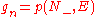This arises due to the constraint of putting N quanta into a state ket where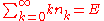and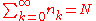, which are the same constraints as in integer partition.

### Example: 3D isotropic harmonic oscillator

The Schrödinger equation of a spherically-symmetric three-dimensional harmonic oscillator can be solved explicitly by separation of variables, see this article for the present case. This procedure is analogous to the separation performed in the hydrogen-like atom problem, but with the spherically symmetric potential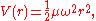whereis the mass of the problem. (Because m will be used below for the magnetic quantum number, mass is indicated by, instead of m, as earlier in this article.)

The solution reads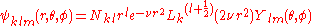where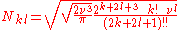is a normalization constant.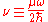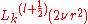are generalized Laguerre polynomials. The order k of the polynomial is a non-negative integer.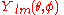is a spherical harmonic function
Spherical harmonics
In mathematics, spherical harmonics are the angular portion of a set of solutions to Laplace's equation. Represented in a system of spherical coordinates, Laplace's spherical harmonics Y_\ell^m are a specific set of spherical harmonics that forms an orthogonal system, first introduced by Pierre...

.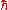is the reduced Planck constant
Planck constant
The Planck constant , also called Planck's constant, is a physical constant reflecting the sizes of energy quanta in quantum mechanics. It is named after Max Planck, one of the founders of quantum theory, who discovered it in 1899...

: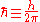.

The energy eigenvalue is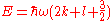The energy is usually described by the single quantum number
Quantum number
Quantum numbers describe values of conserved quantities in the dynamics of the quantum system. Perhaps the most peculiar aspect of quantum mechanics is the quantization of observable quantities. This is distinguished from classical mechanics where the values can range continuously...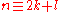Because k is a non-negative integer, for every even n we have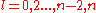and for every odd n we have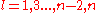. The magnetic quantum number m is an integer satisfying, so for every n and l there are 2l+1 different quantum states, labeled by m. Thus, the degeneracy at level n is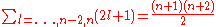where the sum starts from 0 or 1, according to whether n is even or odd.
This result is in accordance with the dimension formula above.

## Coupled harmonic oscillators

In this problem, we consider N equal masses which are connected to their neighbors by springs, in the limit of large N. The masses form a linear chain in one dimension, or a regular lattice in two or three dimensions.

As in the previous section, we denote the positions of the masses by x1, x2, ..., as measured from their equilibrium positions (i.e. xk = 0 if particle k is at its equilibrium position.) In two or more dimensions, the xs are vector
Vector space
A vector space is a mathematical structure formed by a collection of vectors: objects that may be added together and multiplied by numbers, called scalars in this context. Scalars are often taken to be real numbers, but one may also consider vector spaces with scalar multiplication by complex...

quantities. The Hamiltonian of the total system is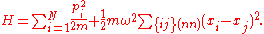The potential energy is summed over "nearest-neighbor" pairs, so there is one term for each spring.

Remarkably, there exists a coordinate transformation to turn this problem into a set of independent harmonic oscillators, each of which corresponds to a particular collective distortion of the lattice. These distortions display some particle-like properties, and are called phonon
Phonon
In physics, a phonon is a collective excitation in a periodic, elastic arrangement of atoms or molecules in condensed matter, such as solids and some liquids...

s. Phonons occur in all (even amorphous
Amorphous solid
In condensed matter physics, an amorphous or non-crystalline solid is a solid that lacks the long-range order characteristic of a crystal....

) solid
Solid
Solid is one of the three classical states of matter . It is characterized by structural rigidity and resistance to changes of shape or volume. Unlike a liquid, a solid object does not flow to take on the shape of its container, nor does it expand to fill the entire volume available to it like a...

s and are extremely important for understanding many of the phenomena studied in solid state physics.

• Quantum machine
Quantum machine
A quantum machine is a human-made device whose collective motion follows the laws of quantum mechanics. The idea that macroscopic objects may follow the laws of quantum mechanics dates back to the advent of quantum mechanics in the early 20th century. However, as highlighted by the Schrödinger's...

• Gas in a harmonic trap
Gas in a harmonic trap
The results of the quantum harmonic oscillator can be used to look at the equilibrium situation for a quantum ideal gas in a harmonic trap, which is a harmonic potential containing a large number of particles that do not interact with each other except for instantaneous thermalizing collisions...

• Creation and annihilation operators
Creation and annihilation operators
Creation and annihilation operators are mathematical operators that have widespread applications in quantum mechanics, notably in the study of quantum harmonic oscillators and many-particle systems. An annihilation operator lowers the number of particles in a given state by one...

• Coherent state
Coherent state
In quantum mechanics a coherent state is a specific kind of quantum state of the quantum harmonic oscillator whose dynamics most closely resembles the oscillating behaviour of a classical harmonic oscillator...

• Morse potential
Morse potential
The Morse potential, named after physicist Philip M. Morse, is a convenient model for the potential energy of a diatomic molecule. It is a better approximation for the vibrational structure of the molecule than the quantum harmonic oscillator because it explicitly includes the effects of bond...

• Hooke's atom
Hooke's atom
Hooke's atom, also known as harmonium, refers to an artificial helium-like atom where the Coulombic electron-nucleus interaction potential isreplaced by a harmonic potential...

• Bertrand's theorem
• Molecular vibration